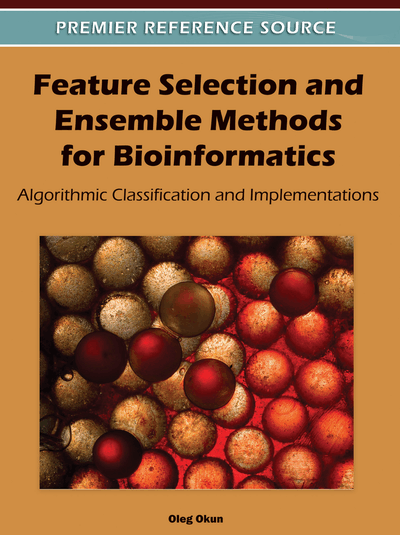# Ensembles of Classifiers

Oleg Okun (Malmö, Sweden)
DOI: 10.4018/978-1-60960-557-5.ch016
Available
\$37.50
No Current Special Offers

Top

## Ensemble Learning

So far in the book, a single algorithm was assumed sufficient for data classification, which means that a single hypothesis was used to make predictions. However, no single algorithm can be superior in all possible cases. This understanding gave rise to the idea of ensemble learning, where a collection, or ensemble, of classifiers is used. Each ensemble member classifies the input data and individual predictions are then combined by a special algorithm, called a combiner, to form the prediction of the whole ensemble. For instance, several hundreds of decision trees can be generated from the same training data, each of them votes for a certain class, and all votes are then fused by a chosen combination rule to produce the final vote for a test instance. The example of a popular combination rule is the ordinary majority voting, where the winning class is determined by the simple majority. If the number of classifiers in the ensemble is 500, then for the two-class problems it is sufficient to have at least 251 votes for class C1 in order for the ensemble to decide in favor of this class. If the number of votes for C1 is less than 250, then it is obvious that another class, C2, got more votes and the final classification is C2. In case when votes split evenly, i.e. 250 for each class, random tie break is usually applied, which is equivalent to generating a uniform number between 0 and 1 and to deciding on C1 if that number is less than or equal to 0.5; otherwise the decision is C2.

The main motivation of using ensembles is to obtain smaller classification error than in the case of any single classifier. Suppose that each classifier hi has an error p – that is, the probability that a randomly chosen instance is misclassified by hi is p. Furthermore, suppose that the errors made by classifiers are independent. In this case, if p is small, then the probability of a large number of misclassifications is very small. For example, an ensemble of five classifiers with p=0.1 (1 error in 10 cases) for each classifier will have an error rate of less than 1 in 100 (Russell & Norvig, 2003)1. Although the independence assumption is often difficult to observe in practice, if predictions of different classifiers in an ensemble are at least a little bit different, thereby reducing the correlation between classification errors, then ensemble learning can be very useful.

Another argument in favor of ensembles is that a group of classifiers is capable of learning a more complex decision boundary separating different classes than a single classifier. This is illustrated in Figure 1, where three classifiers can correctly assign class labels to the solid black circle instances inside a triangular region, while labeling correctly all the open circle instances outside this region. None of the three classifiers can do this alone.

However, you, my ever-watchful readers, may comment: the more complex decision boundary a classifier can learn, the more such a classifier can be affected by overfitting. And you are absolutely right.

If a single classifier may be prone to data overfitting, how about an ensemble, which is a more complex model than a single classifier? Overfitting can be mitigated, though not entirely avoided, if classifiers composing an ensemble are not over-trained (Duin, 2002). If a data set is small, like in the case of microarray gene expression data sets, then the recommendation is not to use trainable combiners as simple majority voting (non-trainable combiner) may often be highly competitive with respect to more sophisticated combination schemes that require training and hence, extra data.

## Complete Chapter List

Search this Book:
Reset